×
Log in to StudySoup
Get Full Access to UVM - Cs 224 - Study Guide
Join StudySoup for FREE
Get Full Access to UVM - Cs 224 - Study Guide

Already have an account? Login here
×
Reset your password

UVM / Computer Science and Engineering / CS 224 / What is a polynomial-time algorithm?

# What is a polynomial-time algorithm? Description

##### Uploaded: 03/23/2016
9 Pages 44 Views 4 Unlocks
Reviews

Zhaojun Lin (Rating: )

CS224 Midterm Exam Study Guide

## What is polynomial time algorithm?The emphasis of the exam is on the concept and intuition and the review of topics studied in  class. Review lecture slides and reinforce the understanding from textbook. Textbook sections  relevant to each topic are shown in parentheses at the end of the topic.

Chapter 2. Basics of Algorithm Analysis

o Justify the argument that an algorithm is efficient if its running time complexity can  be expressed as a polynomial function of the problem size. (2.1)

o A poly-time algorithm holds the desirable scaling property. There exists  constants c > 0 and d > 0 such that on every input of size N, its running time is  bounded by cNd steps. Don't forget about the age old question of Aggregate expenditure represents what?

o Justification: Using a poly-time algorithm works in practice because the  algorithms people develop almost always have small constants (c) and small  exponents (d).

## How is adjacency matrix calculated?Don't forget about the age old question of What is oxidative organisms?

o A problem is “computationally tractable” if there is a worst-case poly-time  algorithm that solves the problem

o Clarify the distinction among the running time complexities expressed in big-Oh  (O), bigomega (Ω), and theta (Ө) notations. (2.2)  We also discuss several other topics like How to understand the concept of socialization?

o Big-Oh(O) – Upper bound; running time can reach no greater than O.

o Bigomega (Ω) – Lower bound of running time.

o Theta (Ө) – Tight-bound; running time is no greater and no less than Ө. o Formal definition and proof of running time complexities. (2.2)

Formal Definitions

o Big-Oh: T(n) = O(f(n)) if there exist constants c > 0 and n0 ≥ 0 such that for all n ≥ n0 we have T(n) ≤ c · f(n).

## How do you calculate run time complexity?o Bigomega: T(n) = Ω(f(n)) if there exist constants c > 0 and n0 ≥ 0 such that for all n  ≥ n0 we have T(n) ≥ c · f(n).

o Theta: T(n) = Θ(f(n)) if T(n) is both O(f(n)) and Ω(f(n)).

Proofs

(2.5) Let k be a fixed constant, and let f1, f2, . . . , fk and h be functions such that fi =  O(h) for all i. Then f1 + f2 + . . . + fk = O(h).  Don't forget about the age old question of What are the 6 drivers of credibility?

Let f be a polynomial of degree d, in which the coefficient ad is positive. Then f = O(nd).

Proof. We write f = a0 + a1 n + a2n2 + . . . + adnd, where ad>0. The upper bound is a  direct application of (2.5). First, notice that coefficients aj for j < d may be negative, but  in any case we have ajnj ≤ |aj|nd for all n ≥ 1. Thus each term in the polynomial is O(nd).

Since f is a sum of a constant number of functions, each of which is O(nd), it follows  from (2.5) that f is O(nd).

o Analyze an algorithm to derive its asymptotic running time complexity. (2.2)

Example: Deriving asymptotic run time of Breadth First Search algorithm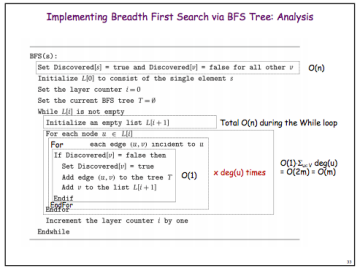Total = O(n) + O(m) = O(m + n) If you want to learn more check out What is extended problem-solving?

Chapter 3. Graphs We also discuss several other topics like How do you find the marginal cost?

o Graph representation: adjacency list, adjacency matrix, and their comparison.

Adjacency List

o Checking if (u, v) is an edge takes O(deg(u)), where deg(u) is the number of  neighbors of u

o Identifying all edges takes Θ(m + n)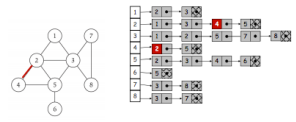Adjacency Matrix

o Checking if (u, v) is an edge takes Θ(1)

o Identifying all edges takes Θ(n2)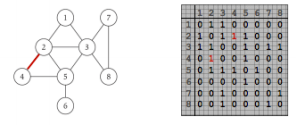o Graph connectivity: completely connected graph, minimally connected graph. The  relationship between the number of edges and the number of vertices in each of the two.

Minimally Connected Graph: A graph with the smallest amount of edges needed to  create a path to every node; the removal of a single edge would leave a disconnected  graph

Number of Edges: n – 1, where n is the number of nodes/vertices

Completely Connected Graph: A graph in which each pair of vertices is directly  connected by an edge.

Number of Edges:

o For undirected: n(n-1)/2, where n is the number of nodes

o For directed: n(n-1), where n is the number of nodes

o Analyze the running time complexity of breadth-first search algorithm when a graph is  represented using an adjacency list or an adjacency matrix. (3.2)

BFS with adjacency list: O(m + n)

o It takes O(n) for the initialization (the first line).

o There are at most n lists L[i] to set up, so initializing an empty list L[i+1]  takes total O(n) time during the execution of the While loop.

o The part “If Discovered[v]…” takes O(1).

o Each node u is visited exactly once during the execution of the While  loop, and for each node, there are deg(u) incident nodes.

o So, the total run-time of the While loop is proportional to the summation  of deg(u) over all nodes, which equals Σu∈V deg(u) = O(2m) = O(m). o So, total O(m+n).

BFS with adjacency matrix: O(n2)

o It takes O(n) for the initialization (the first line).

o There are at most n lists L[i] to set up, so initializing an empty list L[i+1]  takes total O(n) time during the execution of the While loop.

o The part “If Discovered[v]…” takes O(1).

o Each node u is visited exactly once during the execution of the While  loop, and for each node, there are n nodes to check.

o So, the total run-time of the While loop is proportional to n2

o So, total O(n2) + O(n) = O(n2).

o Give an argument for or against building a recursive algorithm for BFS. (3.2)

Because BFS most often uses a queue (FIFO) data structure to keep track of  visited nodes, and it involves observing one layer of nodes at a time, a recursive  algorithm would not be ideal. A recursive algorithm would make more sense for  DFS, which has a recursive nature of searching as deep as possible before  returning back.

o Give the key distinction between the queue structure used for BFS and DFS. (3.2)

BFS uses a FIFO queue structure, which pushes elements out of the queue in the  order they were added, while DFS uses a LIFO queue (aka stack) that pushes  most recently added elements out first.

o Outline the bipartite testing algorithm based on the BFS algorithm and show its  correctness (3.4)

Lines in bold have been added to the BFS algorithm to test for bipartiteness. Algorithm:

// Set Discovered[s] = true and Discovered[v] = false for all other v

// Set Color[s] = red

// Initialize L to consist of the single element s

// Set the layer counter i = 0

// Set the current BFS tree T = ∅

// While L[i] is not empty

// Initialize an empty list L[i +1]

// For each node u in L[i]

// For each edge (u,v) incident to u

// If Discovered[v] = false, then

// Set Discovered[v] = true

// Add edge (u,v) to tree T

// Add v to list L[i +1]

// If i+1 is odd, set Color[v] = blue

// Else, set Color[v] = red

// End If

// End For

// End For

// Increment layer counter i by one

// End While

// Scan all edges and determine whether there is any edge that has the same  color on both end-nodes

Correctness:

By assigning blue to odd numbered layers and red to even numbered layers, we  are essentially saying, only the nodes in those layers can have those respective  colors. Thus, if we find that a particular node has been assigned the same color  as a node in a preceding or following layer, this will indicate that these nodes  belong to an odd numbered cycle, which means we have identified our graph is  bipartite.

o Outline the strong-connectivity testing algorithm using BFS and show the running  time complexity. (3.5)

Algorithm

// Pick any node s.

// Run BFS from s in G.

// Build Grev (reverse the direction of every edge in G).

// Run BFS from s in Grev (set Discovered[v] back to false each time node v is discovered) // Return true iff every node is reachable from s in both BFS in G and BFS in Grev (if  Discovered[] is empty)

Running Time Complexity: O(m + n)

Explanation:

o Each BFS step takes O(m + n).

o Building Grev can be done in O(m + n).

o Traverse all edges in adjacency list exactly once to construct new

adjacency list with traversed edges. (Mark end of each list so adding new  node takes constant time).

o Scanning Discovered array to find if any vertices are left over takes O(n). o Total time: O(3m + 3n + n) = O(m + n).

o Outline the topological sorting algorithm and show the running time complexity. (3.6)  Algorithm

// Maintain count[] for remaining number of incoming edges to node w // Maintain set S of remaining nodes with no incoming edge

// Initialize count[w] for each node w and initialize S

// Repeat until S is empty {

// Remove one node v from S.

// Decrement count[w] for every w with an incoming edge from v,

// and add w to S if count[w] has become 0.

}

Running Time Complexity: O(m + n) (with G in adjacency list)

o Initializing count[w] for every w requires scanning through adjacency  list which takes O(m + n).

o Removing each node exactly once is executed exactly n times (for n

nodes) so takes O(n).

o Decrementing count[w] for every w with incoming edge from v takes the  number of outgoing edges from v for each iteration which totals to O(m),  since there are m edges in total.

o Total: O(2m + 2n) = O(m + n).

Chapter 4. Greedy Algorithms

o State why a greedy algorithm does not always guarantee an optimal solution.

Greedy algorithms are not fit for every situation but work well when a greedy choice can  be made at every stage of a problem and when a solution for part of the problem is also a  solution to the entire problem. Sometimes, there are algorithms that prove to be more  optimal than a greedy strategy, but it all depends on the given problem.

o For each of the following problems, state the optimal greedy strategy and justify its  optimality, and find an optimal solution given a problem instance; given a greedy  strategy, prove or disprove if it is an optimal strategy:

o Interval scheduling (4.1)

Earliest finish time

o Interval partitioning (4.1)

Earliest start time

o Minimum lateness (4.2)

Earliest deadline

o State the greedy strategy of the Dijkstra’s shortest path algorithm and justify why it  ensures an optimal solution. (4.5)

Start at the source node s and follow the path to t that chooses each edge, which  minimizes the total distance from s to t.

o Show the shortest paths as they are constructed during the execution of the  Dijkstra’s algorithm. (4.5)

Example: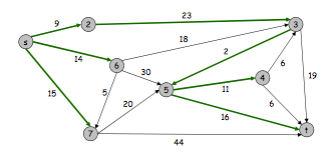o State the similarity and dissimilarity between the Dijkstra’s shortest path algorithm  and the Prim’s minimum spanning tree algorithm. (4.5)

While both algorithms are concerned with finding a shortest path, Dijkstra’s shortest path  algorithm is used to find the shortest path between two nodes, s and t, but is not  necessarily concerned with hitting every node if it does not have to in order to reach t,  while Prim’s is a MST algorithm, that finds the shortest path which touches every node in  the graph. In addition, Dijkstra’s evaluates the total weighted distance from s to a node as it considers which node to add to the cloud next, while Prim’s is only concerned with  the individual weights of each edge (u,v) leading from a node u, and it considers whether  or not adding node v will create a cycle.

o For each of the Prim’s and Kruskal’s minimum spanning tree algorithms, state the  greedy strategy and justify its optimality.

Kruskal’s

Greedy Strategy:

Starting with the smallest weighted edge in a graph, continually add the next  smallest weighted edge to T if it will not create a cycle.

Optimality: For each edge considered:

Case 1: If adding e to T creates a cycle, then e is discarded. This is justified by  the cycle property because e has the largest weight among all edges in the cycle.

Case 2: Otherwise, add e = (u, v) to T. This is justified by the cut

property, where the cut S is the set of nodes in u's connected

component, because, since adding e to T does not create a cycle, e

has the smallest weight among all edges remaining to be added from

the cutset of S.

Prim’s

Greedy Strategy:

Start at any node s and add to cut S, continually consider the smallest weighted  edge in the cutset of S, while adding the newly explored node to S.

Optimality: Justified by the cut property: Let S be any cut in a graph, and let e  be the minimum weighted edge in the cutset D of S. Then the MST contains e.

o Describe briefly how the Kruskal’s minimum spanning tree algorithm can be  implemented using the union-find data structure. (4.5)

You can implement Kruskal’s algorithm using a forest implementation of disjoint sets.  Initialize all nodes in the graph as disjoint singleton sets. Each time a new edge (u,v) is  considered, check to see if u and v are equivalent (have the same root node) by calling  find(u) and find(v). If they return different values, this means adding this edge would not  create a cycle, so union them. Now they are apart of the same tree and next time find is  called on either one, the same root node will be returned. Continue this process until all

nodes belong to the same set; then you know you’ve reached every node and the MST is  complete.

o Show the minimum spanning tree as it is constructed during the execution of the  Prim’s or the Kruskal’s algorithm. (4.5)

Examples: Numbers in red indicate order the edge was added to MST

1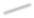3 1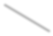26

5 4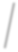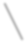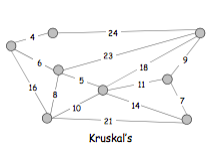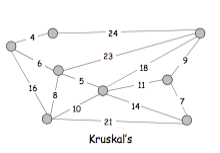7

1

3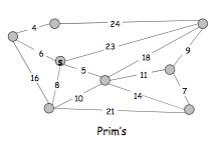7

1 2 4

5

6

Chapter 5. Divide and Conquer. (Do not use Master theorem when solving a recurrence  relation.)

o Describe the divide-and-conquer approach in algorithm design.

o Break up problem into several parts

o Solve each part recursively

o Combine solutions as parts in overall solution

o State the approach to deriving the running time complexity of a divide-and-conquer  algorithm.

Building and solving a recurrence relation.

o Outline the mergesort algorithm using divide-and-conquer and derive the running  time complexity. (5.1)

Algorithm

// Divide array into two halves

// Recursively sort each half

// Merge two sorted halves to make one sorted whole

Running Time Complexity: O(nLogn)

Runtime T(n)

Divide O(1)

Sort 2T(n/2)

Merge O(n)

(Using pre-sorted arrays and temporary array)

T(n) ≤ T (⎣n/2⎦)+ T (⎡n/2⎤)+ O(n) => T(n) = O(n log n)

o Outline the counting inversion algorithm using divide-and-conquer and derive the  running time complexity. (5.3)

Algorithm

// Divide array into two halves

// Recursively count inversion in each half

// Count inversions between the two halves, while merging into whole and return  sum of all three counts (Make sure two halves are pre-sorted, and use auxiliary  array)

Running Time Complexity: O(nLogn)

Runtime T(n)

Divide O(1)

Sort 2T(n/2)

Merge O(n)

(Using pre-sorted arrays and auxiliary array)

T(n) ≤ T (⎣n/2⎦)+ T (⎡n/2⎤)+ O(n) => T(n) = O(n log n)

o Outline the Karatsuba’s integer multiplication algorithm using divide-and-conquer  and derive the running time complexity. (5.5)

Multiplying 2 n-digit integers Algorithm

// Add two ½ n digit integers

// Multiple three ½ n digit integers

// Add, subtract, and shift ½ n digit integers to obtain result

Running Time Complexity: O(n1.585)

Recursive calls Add, subtract, shift

Page ExpiredIt looks like your free minutes have expired! Lucky for you we have all the content you need, just sign up here